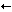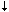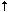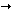home | O'Reilly's CD bookshelfs | FreeBSD | Linux | Cisco | Cisco Exam

#Chapter 8The vi Editor## 8.2 Movement Commands

A number preceding a command repeats the movement. Movement commands are also objects for change, delete, and yank operations.

### 8.2.1 Character

 ```h ``` , ```j ``` , ```k ``` , ```l ``` Left, down, up, right (,,,). ```Spacebar ``` Right.

### 8.2.2 Text

 ```w ``` , ```W ``` , ```b ``` , ```B ``` Forward, backward by word. ```e ``` , ```E ``` End of word. ```) ``` , ```( ``` Beginning of next, current sentence. ```} ``` , ```{ ``` Beginning of next, current paragraph. ```]] ``` , ```[[ ``` Beginning of next, current section.

### 8.2.3 Lines

 ```0 ``` , ```\$ ``` First, last position of current line. ```^ ``` First nonblank character of current line. ```+ ``` , ```- ``` First character of next, previous line. ```Return ``` First character of next line. n ```| ``` Column n of current line. ```H ``` Top line of screen. ```M ``` Middle line of screen. ```L ``` Last line of screen. n ```H ``` n lines after top line. n ```L ``` n lines before last line.

### 8.2.4 Screens

 ```CTRL-F ``` Scroll forward, backward one screen. ```CTRL-B ``` ```CTRL-D ``` Scroll down, up one-half screen. ```CTRL-U ``` ```CTRL-E ``` Show one more line at bottom, top of window. ```CTRL-Y ``` ```z ``` Return Reposition line with cursor to top of screen. ```z. ``` Reposition line with cursor to middle of screen. ```z- ``` Reposition line with cursor to bottom of screen. ```CTRL-L ``` Redraw screen (without scrolling). ```CTRL-R ```

### 8.2.5 Searches

 ```/ ``` text Search forward for text . ```n ``` Repeat previous search. ```N ``` Repeat search in opposite direction. ```/ ``` Repeat forward search. ```? ``` Repeat previous search backward. ```? ``` text Search backward for text . ```/ ``` text ```/+ ``` n Go to line n after text . ```? ``` text ```?- ``` n Go to line n before text . ```% ``` Find match of current parenthesis, brace, or bracket. ```f ``` x Move search forward to x on current line. ```F ``` x Move search backward to x on current line. ```t ``` x Search forward to character before x in current line. ```T ``` x Search backward to character after x in current line. ```, ``` Reverse search direction of last ```f ``` , ```F ``` , ```t ``` , or ```T ``` . ```; ``` Repeat last character search (```f ``` , ```F ``` , ```t ``` , or ```T ``` ).

### 8.2.6 Line Numbering

 ```CTRL-G ``` Display current line number. n ```G ``` Move to line number n . ```G ``` Move to last line in file. ```: ``` n Move to line number n .

### 8.2.7 Marking Position

 ```m ``` x Mark current position with character x . ```` ``` x Move cursor to mark x . ```' ``` x Move to start of line containing x . ````` ``` Return to previous mark (or to location prior to a search). ```'' ``` Like above, but return to start of line.8.1 Review of vi Operations8.3 Edit Commands